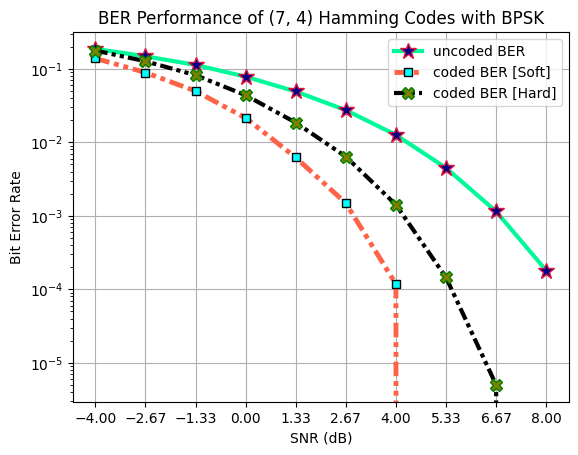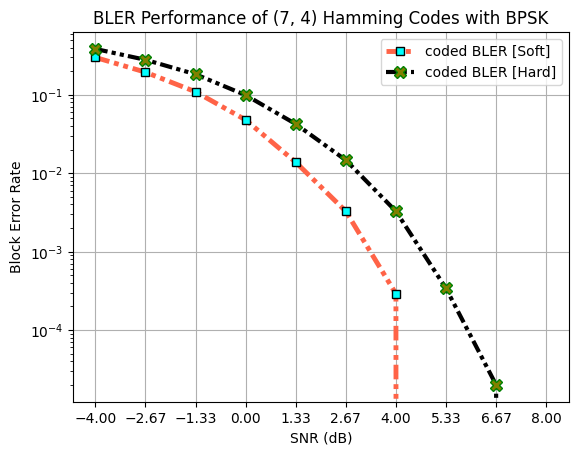# Hamming Codes

Hamming codes are perfect codes for (k,n) when $$k = 2^r-r-1$$, $$n = 2^r-1$$ where $$r$$ is the number of parity bits. The code-rate ($$R = \frac{k}{n} = \frac{2^r-r-1}{2^r-1} = 1 - \frac{r}{2^r-1}$$) of Hamming codes increases as $$r$$ increases. Hamming codes can detect $$1$$ or $$2$$ bit errors and can correct $$1$$ bit error.

Table of Content:

## Import Libraries

### Python Libraries

:

import os
os.environ["CUDA_VISIBLE_DEVICES"] = "-1"
os.environ['TF_CPP_MIN_LOG_LEVEL'] = '3'

import numpy as np
# %matplotlib widget
import matplotlib.pyplot as plt


### 5G Toolkit Libraries

:

from toolkit5G.SymbolMapping import Demapper
from toolkit5G.SymbolMapping import Mapper
from toolkit5G.ChannelCoder  import HammingEncoder
from toolkit5G.ChannelCoder  import HammingDecoder


## Hamming Codes Parameters

• m is number of parity check bits

• k is number of information bits

• n is size of the codeword

:

## Hamming Code Configurations
m = 3
k = 2**m - m - 1
n = 2**m - 1


## Simulation Setup

• numBatches defines numBatches.

• bits information bits.

• encBits encoded bits.

• constellation_type constellation type.

• num_bits_per_symbol number of bits per symbols.

• demapping_method demapping methods.

• hard_out if true symbol demapper return hard bits (0/1) otherwise log likelihood ratios are returned.

Note: For hard outputs, the syndrome based Hamming decoder is used. On the other hand for the soft outputs, the sphere decoder is used.

:

## Payload Generation
numBatches = 10000000
bits       = np.random.randint(2, size = (numBatches, k))

## Hamming Encoder
encBits = HammingEncoder(k,n)(bits)

## Rate Matching (No rate matching as of now)
codeword = encBits

## Symbol Mapping
constellation_type  = "bpsk"
num_bits_per_symbol = 1
mapperObject        = Mapper(constellation_type, num_bits_per_symbol)
symbols             = mapperObject(codeword)

SNRdB = np.linspace(-4,8,10)
SNR   = 10**(SNRdB/10)

uncodedBER    = np.zeros(SNR.shape)
codedBERhard  = np.zeros(SNR.shape)
codedBERsoft  = np.zeros(SNR.shape)
codedBLERhard = np.zeros(SNR.shape)
codedBLERsoft = np.zeros(SNR.shape)

## Symbol Demapping
demapping_method = "app"
hard_out         = False

demapper         = Demapper(demapping_method, constellation_type,
num_bits_per_symbol, hard_out = hard_out)
snrIndex = 0
for snr in SNR:

# AWGN Channel
symbs   = symbols + np.sqrt(0.5/snr)*(np.random.standard_normal(size=symbols.shape)+1j*np.random.standard_normal(size=symbols.shape)).astype(np.complex64)

# Symbol Demapping
llrEst  = demapper([symbs, np.float32(1/snr)])

# Decoding based on Hard Inputs
uncBits = np.where(llrEst > 0, np.int8(1), np.int8(0))
uncodedBER[snrIndex] = np.mean(np.abs(uncBits-encBits))
decoder = HammingDecoder(k,n)
decBits = decoder(uncBits)

# BER and BLER for decoding based on Hard Inputs
codedBERhard[snrIndex] = np.mean(np.abs(bits-decBits))
codedBLERhard[snrIndex]= np.mean(np.where(np.sum(np.abs(bits-decBits), axis=1)>0, True, False))

# Decoding based on Soft Inputs
decoder = HammingDecoder(k,n)
decBits = decoder(llrEst, "sphereDecoding")

# BER and BLER for decoding based on Soft Inputs
codedBERsoft[snrIndex] = np.mean(np.abs(bits-decBits))
codedBLERsoft[snrIndex]= np.mean(np.where(np.sum(np.abs(bits-decBits), axis=1)>0, True, False))

print("At SNR(dB): "+str(SNRdB[snrIndex])+" | coded BER: "+str(codedBERhard[snrIndex])+" | uncoded BER: "+str(uncodedBER[snrIndex]))

snrIndex += 1

At SNR(dB): -4.0 | coded BER: 0.1774301 | uncoded BER: 0.18609175714285714
At SNR(dB): -2.666666666666667 | coded BER: 0.127843825 | uncoded BER: 0.14910897142857144
At SNR(dB): -1.3333333333333335 | coded BER: 0.081641725 | uncoded BER: 0.11257671428571428
At SNR(dB): 0.0 | coded BER: 0.044168875 | uncoded BER: 0.07864995714285715
At SNR(dB): 1.333333333333333 | coded BER: 0.01914105 | uncoded BER: 0.0495807
At SNR(dB): 2.666666666666666 | coded BER: 0.0061892 | uncoded BER: 0.027259314285714285
At SNR(dB): 4.0 | coded BER: 0.001367925 | uncoded BER: 0.012492042857142857
At SNR(dB): 5.333333333333332 | coded BER: 0.000177875 | uncoded BER: 0.004495342857142857
At SNR(dB): 6.666666666666666 | coded BER: 1.205e-05 | uncoded BER: 0.0011498857142857144
At SNR(dB): 8.0 | coded BER: 1.25e-07 | uncoded BER: 0.00019195714285714287


## Performance Evaluation: SNR vs BER

Following plots demonstate the BER vs SNR performance of following:

• Uncoded transmission (Hamming Code is not used)

• Hamming decoder (Syndrome based decoder) taking hard bits (0/1) as inputs.

• Hamming decoder (Sphere decoder) taking soft bits (log likelihood ratios) as inputs.

:

fig, ax = plt.subplots()

ax.semilogy(SNRdB, uncodedBER,   'mediumspringgreen', lw = 3, linestyle = "solid",
marker = "*", ms = 12, mec = "crimson", mfc = "darkblue", label = "uncoded BER")
ax.semilogy(SNRdB, codedBERsoft, 'tomato', lw = 3.5, linestyle = (0, (3, 1, 1, 1, 1, 1)),
marker = "s", ms = 6, mec = "k", mfc = "cyan", label = "coded BER [Soft]")
ax.semilogy(SNRdB, codedBERhard, 'k', lw = 3, linestyle = (0, (3, 1, 1, 1, 1, 1)),
marker = "X", ms = 9, mec = "green", mfc = "olive", label = "coded BER [Hard]")
ax.legend()
ax.set_xticks(SNRdB)
ax.grid()
ax.set_ylabel("Bit Error Rate")
ax.set_xlabel("SNR (dB)")
ax.set_title("BER Performance of ("+str(n)+", "+str(k)+") Hamming Codes with BPSK")
plt.show()## Performance Evaluation: SNR vs BLER

Following plots demonstate the BLER vs SNR performance of following:

• Hamming decoder (Syndrome based decoder) taking hard bits (0/1) as inputs.

• Hamming decoder (Sphere decoder) taking soft bits (log likelihood ratios) as inputs.

:

fig, ax = plt.subplots()

ax.semilogy(SNRdB, codedBLERsoft, 'tomato', lw = 3.5, linestyle = (0, (3, 1, 1, 1, 1, 1)),
marker = "s", ms = 6, mec = "k", mfc = "cyan", label = "coded BLER [Soft]")
ax.semilogy(SNRdB, codedBLERhard, 'k', lw = 3, linestyle = (0, (3, 1, 1, 1, 1, 1)), marker = "X",
ms = 9, mec = "green", mfc = "olive", label = "coded BLER [Hard]")
ax.legend()
ax.set_xticks(SNRdB)
ax.grid()
ax.set_ylabel("Block Error Rate")
ax.set_xlabel("SNR (dB)")
ax.set_title("BLER Performance of ("+str(n)+", "+str(k)+") Hamming Codes with BPSK")
plt.show()## Conclusions

• The Hamming decoder with soft inputs outputs the rest of the techniques for both BER as well as BLER.

• Sphere Decoding has very high complexity which scales exponentially with parity bits (m).

[ ]: